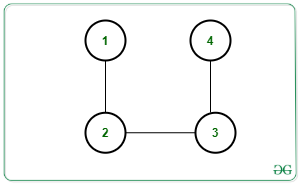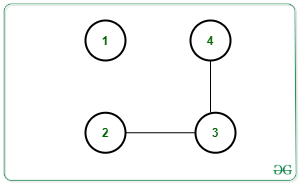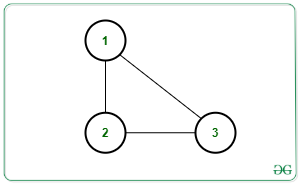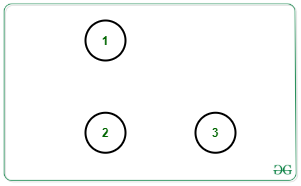# Maximum number of edges to be removed to contain exactly K connected components in the Graph

• Difficulty Level : Hard
• Last Updated : 27 Aug, 2021

Given an undirected graph G with N nodes, M edges, and an integer K, the task is to find the maximum count of edges that can be removed such that there remains exactly K connected components after the removal of edges. If the graph cannot contain K connect components, print -1.

Examples:

Input: N = 4, M = 3, K = 2, Edges[][] = {{1, 2}, {2, 3}, {3, 4}}Output: 1
Explanation:
One possible way is to remove edge [1, 2]. Then there will be 2 connect components as shown below:Input: N = 3, M = 3, K = 3, Edges[][] = {{1, 2}, {2, 3}, {3, 1}}Output: 3
Explanation: All edges can be removed to make 3 connected components as shown below:Approach: To solve the given problem, count the number of connected components present in the given graph. Let the count be C. Observe that if C is greater than K then no possible edge removal can generate K connected components as the number of connected components will only increase. Otherwise, the answer will always exist.

Following observations need to be made in order to solve the problem:

• Suppose C1, C2, …, Cc, are the number of node in each connected component. Then, each component must have edges as C1 – 1, C2 – 1, …, C-1 after edges are removed. Therefore,

C1 – 1 + C2 – 1 + … + Cc – 1 = C1 + C2 + … + Cc – C = N – C, where N is the number of nodes.

• The above condition will give us the C connected components by removing M – (N – C) edges as N – C edges are needed to make C components. To get K components, (K – C) more edges must be removed.
• Hence, the total count of edges to be removed is given by:

M – (N – C) + (K – C) = M – N + K

Follow the steps below to solve the problem:

1. Count the number of connected components present in the given graph. Let the count be C.
2. If C is greater than K, print -1.
3. Else print M – N + K where N is the number f nodes, M is the number of edges and K is the required number of connected components.

Below is the implementation of the above approach:

## C++

 `// C++ program for the above approach``#include ``using` `namespace` `std;` `class` `Graph``{``  ``public``:``    ``int` `V;``    ``map<``int``, vector<``int``>> adj;` `    ``Graph(``int``);``    ``void` `addEdge(``int``, ``int``);``    ``void` `DFS(``int``, vector<``bool``> &);``} * g;` `// Constructor``Graph::Graph(``int` `V)``{``  ` `  ``// No. of vertices``  ``this``->V = V;``  ` `  ``// Dictionary of lists``  ``for``(``int` `i = 1; i <= V; i++)``    ``adj[i] = vector<``int``>();``}` `// Function to add edge``// in the graph``void` `Graph::addEdge(``int` `v, ``int` `w)``{``  ``adj[v].push_back(w);``  ``adj[w].push_back(v);``}` `// Function to perform DFS``void` `Graph::DFS(``int` `s, vector<``bool``> &visited)``{``  ` `  ``// Create a stack for DFS``  ``stack<``int``> stack;` `  ``// Push the current source node``  ``stack.push(s);``  ``while` `(!stack.empty())``  ``{``    ` `    ``// Pop a vertex from stack``    ``// and print it``    ``s = stack.top();``    ``stack.pop();` `    ``// Traverse adjacent vertices``    ``// of the popped vertex s``    ``for``(``auto` `node : adj[s])``    ``{``      ``if` `(!visited[node])``      ``{``        ` `        ``// If adjacent is unvisited,``        ``// push it to the stack``        ``visited[node] = ``true``;``        ``stack.push(node);``      ``}``    ``}``  ``}``}` `// Function to return the count``// edges removed``void` `countRemovedEdges(``int` `N, ``int` `M, ``int` `K)``{``  ``int` `C = 0;` `  ``// Initially mark all vertices``  ``// as not visited``  ``vector<``bool``> visited(g->V + 1, ``false``);` `  ``for``(``int` `node = 1; node <= N; node++)``  ``{``    ` `    ``// If node is unvisited``    ``if` `(!visited[node])``    ``{``      ` `      ``// Increment Connected``      ``// component count by 1``      ``C = C + 1;` `      ``// Perform DFS Traversal``      ``g->DFS(node, visited);` `      ``// Print the result``      ``if` `(C <= K)``        ``cout << M - N + K << endl;``      ``else``        ``cout << -1 << endl;``    ``}``  ``}``}` `// Driver Code``int` `main(``int` `argc, ``char` `const` `*argv[])``{``  ``int` `N = 4, M = 3, K = 2;` `  ``// Create Graph``  ``g = ``new` `Graph(N);` `  ``// Given Edges``  ``g->addEdge(1, 2);``  ``g->addEdge(2, 3);``  ``g->addEdge(3, 4);` `  ``// Function Call``  ``countRemovedEdges(N, M, K);``}` `// This code is contributed by sanjeev2552`

## Java

 `// Java program to implement ``// the above approach``import` `java.util.*;``class` `GFG``{` `  ``static` `ArrayList> graph;` `  ``// Function to perform DFS``  ``static` `void` `DFS(``int` `s, ``boolean``[] visited)``  ``{` `    ``// Create a stack for DFS``    ``Stack stack = ``new` `Stack<>();` `    ``// Push the current source node``    ``stack.push(s);``    ``while` `(!stack.isEmpty())``    ``{` `      ``// Pop a vertex from stack``      ``// and print it``      ``s = stack.peek();``      ``stack.pop();` `      ``// Traverse adjacent vertices``      ``// of the popped vertex s``      ``for``(Integer node : graph.get(s))``      ``{``        ``if` `(!visited[node])``        ``{` `          ``// If adjacent is unvisited,``          ``// push it to the stack``          ``visited[node] = ``true``;``          ``stack.push(node);``        ``}``      ``}``    ``}``  ``}` `  ``// Function to return the count``  ``// edges removed``  ``static` `void` `countRemovedEdges(``int` `N, ``int` `M, ``int` `K)``  ``{``    ``int` `C = ``0``;` `    ``// Initially mark all vertices``    ``// as not visited``    ``boolean``[] visited = ``new` `boolean``[N+``1``];` `    ``for``(``int` `node = ``1``; node <= N; node++)``    ``{` `      ``// If node is unvisited``      ``if` `(!visited[node])``      ``{` `        ``// Increment Connected``        ``// component count by 1``        ``C = C + ``1``;` `        ``// Perform DFS Traversal``        ``DFS(node, visited);` `        ``// Print the result``        ``if` `(C <= K)``          ``System.out.println(M - N + K);``        ``else``          ``System.out.println(-``1``);``      ``}``    ``}``  ``}` `  ``// Driver code``  ``public` `static` `void` `main (String[] args)``  ``{``    ``int` `N = ``4``, M = ``3``, K = ``2``;` `    ``// Create Graph``    ``graph = ``new` `ArrayList<>();` `    ``for``(``int` `i = ``0``; i <= N; i++)``      ``graph.add(``new` `ArrayList());` `    ``// Given Edges``    ``graph.get(``1``).add(``2``);``    ``graph.get(``2``).add(``3``);``    ``graph.get(``3``).add(``4``);` `    ``// Function Call``    ``countRemovedEdges(N, M, K);``  ``}``}` `// This code is contributed by offbeat.`

## Python3

 `# Python3 program for the above approach` `class` `Graph:` `    ``# Constructor``    ``def` `__init__(``self``, V):` `        ``# No. of vertices``        ``self``.V ``=` `V` `        ``# Dictionary of lists``        ``self``.adj ``=` `{i: [] ``for` `i ``in` `range``(``1``, V ``+` `1``)}` `    ``# Function to add edge``    ``# in the graph``    ``def` `addEdge(``self``, v, w):``        ``self``.adj[v].append(w)``        ``self``.adj[w].append(v)` `    ``# Function to perform DFS``    ``def` `DFS(``self``, s, visited):` `    ``# Create a stack for DFS``        ``stack ``=` `[]` `        ``# Push the current source node``        ``stack.append(s)``        ``while` `(``len``(stack)):` `            ``# Pop a vertex from stack``            ``# and print it``            ``s ``=` `stack[``-``1``]``            ``stack.pop()` `            ``# Traverse adjacent vertices``            ``# of the popped vertex s``            ``for` `node ``in` `self``.adj[s]:``                ``if` `(``not` `visited[node]):` `                    ``# If adjacent is unvisited,``                    ``# push it to the stack``                    ``visited[node] ``=` `True``                    ``stack.append(node)` `# Function to return the count``# edges removed``def` `countRemovedEdges(N, M, K):` `    ``C ``=` `0` `    ``# Initially mark all vertices``    ``# as not visited``    ``visited ``=` `[``False` `for` `i ``in` `range``(g.V ``+` `1``)]` `    ``for` `node ``in` `range``(``1``, N ``+` `1``):` `        ``# If node is unvisited``        ``if` `(``not` `visited[node]):` `            ``# Increment Connected``            ``# component count by 1``            ``C ``=` `C ``+` `1` `            ``# Perform DFS Traversal``            ``g.DFS(node, visited)` `    ``# Print the result``    ``if` `C <``=` `K:``        ``print``(M ``-` `N ``+` `K)``    ``else``:``        ``print``(``-``1``)`  `# Driver Code` `N, M, K ``=` `4``, ``3``, ``2` `# Create Graph``g ``=` `Graph(N)` `# Given Edges``g.addEdge(``1``, ``2``)``g.addEdge(``2``, ``3``)``g.addEdge(``3``, ``4``)` `# Function Call``countRemovedEdges(N, M, K)`

## C#

 `// C# program to implement``// the above approach``using` `System;``using` `System.Collections.Generic;``class` `GFG {``    ` `  ``static` `List> graph;`` ` `  ``// Function to perform DFS``  ``static` `void` `DFS(``int` `s, ``bool``[] visited)``  ``{`` ` `    ``// Create a stack for DFS``    ``Stack<``int``> stack = ``new` `Stack<``int``>();`` ` `    ``// Push the current source node``    ``stack.Push(s);``    ``while` `(stack.Count > 0)``    ``{`` ` `      ``// Pop a vertex from stack``      ``// and print it``      ``s = (``int``)stack.Peek();``      ``stack.Pop();`` ` `      ``// Traverse adjacent vertices``      ``// of the popped vertex s``      ``foreach``(``int` `node ``in` `graph[s])``      ``{``        ``if` `(!visited[node])``        ``{`` ` `          ``// If adjacent is unvisited,``          ``// push it to the stack``          ``visited[node] = ``true``;``          ``stack.Push(node);``        ``}``      ``}``    ``}``  ``}`` ` `  ``// Function to return the count``  ``// edges removed``  ``static` `void` `countRemovedEdges(``int` `N, ``int` `M, ``int` `K)``  ``{``    ``int` `C = 0;`` ` `    ``// Initially mark all vertices``    ``// as not visited``    ``bool``[] visited = ``new` `bool``[N+1];`` ` `    ``for``(``int` `node = 1; node <= N; node++)``    ``{`` ` `      ``// If node is unvisited``      ``if` `(!visited[node])``      ``{`` ` `        ``// Increment Connected``        ``// component count by 1``        ``C = C + 1;`` ` `        ``// Perform DFS Traversal``        ``DFS(node, visited);`` ` `        ``// Print the result``        ``if` `(C <= K)``          ``Console.WriteLine(M - N + K);``        ``else``          ``Console.WriteLine(-1);``      ``}``    ``}``  ``}``  ` `  ``// Driver code``  ``static` `void` `Main() {``    ``int` `N = 4, M = 3, K = 2;`` ` `    ``// Create Graph``    ``graph = ``new` `List>();`` ` `    ``for``(``int` `i = 0; i <= N; i++)``      ``graph.Add(``new` `List<``int``>());`` ` `    ``// Given Edges``    ``graph.Add(2);``    ``graph.Add(3);``    ``graph.Add(4);`` ` `    ``// Function Call``    ``countRemovedEdges(N, M, K);``  ``}``}` `// This code is contributed by rameshtravel07.`

## Javascript

 ``

Output:

`1`

Time Complexity: O(N + M)
Auxiliary Space: O(M + N)

My Personal Notes arrow_drop_up